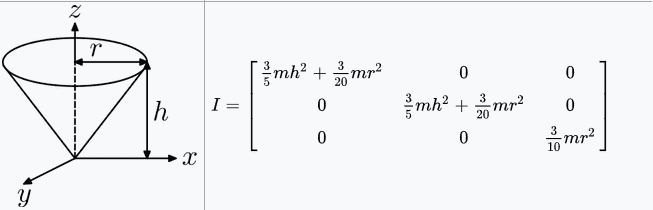# Inertia tensor of cone around its apex

Thread moved from the technical forums, so no Homework Template is shown
Im trying to calculate the principals moments of inertia (Ixx Iyy Izz) for the inertia tensor by triple integration using cylindrical coordinates in MATLAB.Code:
% Symbolic variables
syms r z theta R h M; % R (Radius) h(height) M(Mass)

% Ixx
unox = int((z^2+(r*sin(theta))^2)*r,z,r,h); % First integration
dosx = int(unox,r,0,R); % Second Integration
tresx = int(dosx,theta,0,2*pi); % Third integration

Ix = tresx / ((pi*R^2*h)/3); % Division by the volume of a cone (Mass since densitiy is 1)
Ix = Ix*M  % Setting up in terms of mass M

% Iyy
unoy = int((z^2+(r*cos(theta))^2)*r,z,r,h);
dosy = int(unoy,r,0,R);
tresy = int(dosy,theta,0,2*pi);

Iy = tresy / ((pi*R^2*h)/3);
Iy = Iy*M

% Izz
unoz = int(r^2*r,z,r,h);
dosz = int(unoz,r,0,R);
tresz = int(dosz,theta,0,2*pi);

Iz = tresz / ((pi*R^2*h)/3);
Iz = Iz*M

I plug in test values in both the formulas from the image and the code and they seem correct except from Iz in which the height (h) still appears and terms like 3h-2r instead of h appear, Am I missing something in the integrations? Thank you in advance.

#### Attachments

Orodruin
Staff Emeritus
Homework Helper
Gold Member
Did you try doing the integrals by hand? (They are not difficult.)

•vanhees71
Did you try doing the integrals by hand? (They are not difficult.)

In cylindrical coordinates for a solid cone the only non-trivial boundarie would be on the first integration, since its a solid of revolution, theta goes grom 0 to 2*pi and r goes from 0 to R (radius of cone).
z would go from z = r (x^2 + y^2) to z = h (height of cone)

Orodruin
Staff Emeritus
•